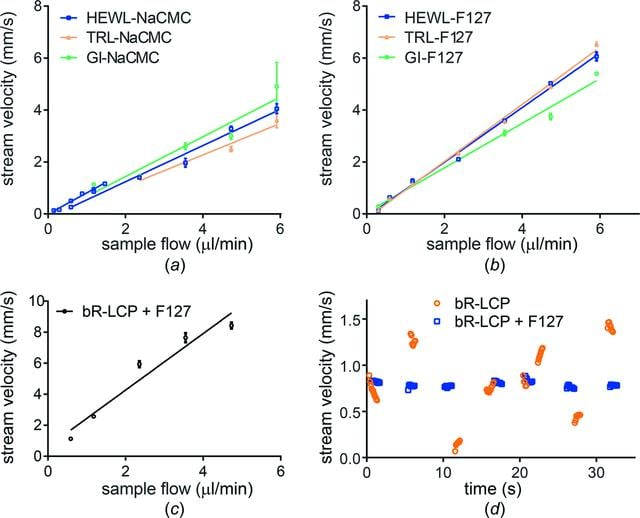disable zoom     view article Figure 1 Measurements of stream velocity and its dependence on the flow rate [parts (a), (b) and (c)] and time [part (d)]. (a), (b), (c) The average velocities at various sample flow rates were plotted and fitted with a simple linear regression. The standard deviation is plotted for all velocity values, but it is not displayed if it is smaller than the size of the symbol. Stream velocities of (a) NaCMC and (b) F-127 as measured for different flow rates for various embedded crystals (GI, HEWL and TRL, represented by green dots, blue squares and yellow triangles, respectively). Embedded HEWL crystals in NaCMC were measured in both small and large sample reservoir injectors, hence the two blue plots covering lower and higher flow rates in part (a). (c) Stream velocity of bR-LCP mixed with F-127 (in a 3+1 ratio) measured at different flow rates. (d) At a constant flow rate (0.3 and 0.35 µl min−1 for the bR-LCP sample without and with F-127, respectively) at intervals of approximately 5 s, 5–10 instantaneous stream velocities were calculated and plotted for both samples. Data points for bR-LCP and bR-LCP with F-127 are represented as orange circles and blue squares, respectively.

IUCrJ
Volume 4| Part 4| July 2017| Pages 400-410
ISSN: 2052-2525